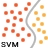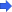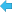Back to Adaptive Vision Library website# SVM_ClassifyMultiple

Classifies input points based on trained model

### Syntax

C++
C#

void avl::SVM_ClassifyMultiple
(
const avl::SvmModel& inSvmModel,
const atl::Array<atl::Array<float> >& inVectorArray,
atl::Array< int >& outPredictions,
atl::Optional<atl::Array< int >& > outModelClasses = atl::NIL,
atl::Optional<atl::Array<atl::Array<float>>& > outClassProbabilities = atl::NIL
)


### Parameters

Name Type Default DescriptioninSvmModel const SvmModel& Input trained modelinVectorArray const Array<Array<float> >& Data vector array of unknown classesoutPredictions Arrayint >& Predicted classesoutModelClasses Optional<Arrayint >& > NIL All known model classes in orderoutClassProbabilities Optional<Array<Array<float>>& > NIL For each data vector the probability of belonging to each class

### Optional Outputs

The computation of following outputs can be switched off by passing value atl::NIL to these parameters: outModelClasses, outClassProbabilities.

### Description

The operation predicts classes for the given data points. It takes an array of data vectors (inVectorArray) as an argument. Each vector has to be of the same size as vectors used for training the model. The operation outputs predicted class (outPredictions) for each data vector.
outModelClasses are all class labels encountered during training.
outClassProbabilities provides, for each vector, estimated probability of this vector belonging to each class. Precisely, in each array the value under index i denotes probability of given vector belonging to the class outModelClasses[i].

### Errors

List of possible exceptions:

Error type Description
DomainError Empty vector array in SVM_ClassifyMultiple.
DomainError Incorrect vector size in SVM_Classify
DomainError Incorrect, uninitialized or not trained SvmModel in SVM_ClassifyMultiple.